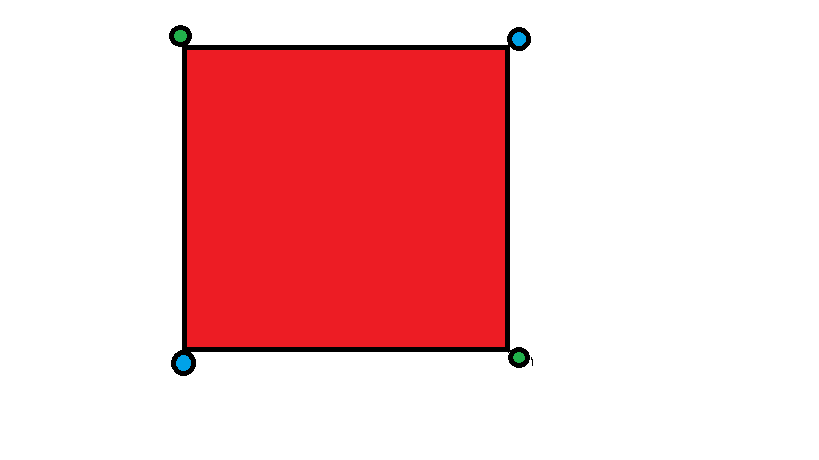# A problem on Coulomb's LawFour charged particles are placed on the vertices of a square. The charges are $+Q$(depicted by green) and $+q$(depicted by blue). What should be $\frac{Q}{q}$ so that both the particles having charge $+Q$ experience net zero electrostatic force?

SwapnilNote by Swapnil Das
4 years, 5 months ago

This discussion board is a place to discuss our Daily Challenges and the math and science related to those challenges. Explanations are more than just a solution — they should explain the steps and thinking strategies that you used to obtain the solution. Comments should further the discussion of math and science.

When posting on Brilliant:

• Use the emojis to react to an explanation, whether you're congratulating a job well done , or just really confused .
• Ask specific questions about the challenge or the steps in somebody's explanation. Well-posed questions can add a lot to the discussion, but posting "I don't understand!" doesn't help anyone.
• Try to contribute something new to the discussion, whether it is an extension, generalization or other idea related to the challenge.

MarkdownAppears as
*italics* or _italics_ italics
**bold** or __bold__ bold
- bulleted- list
• bulleted
• list
1. numbered2. list
1. numbered
2. list
Note: you must add a full line of space before and after lists for them to show up correctly
paragraph 1paragraph 2

paragraph 1

paragraph 2

[example link](https://brilliant.org)example link
> This is a quote
This is a quote
    # I indented these lines
# 4 spaces, and now they show
# up as a code block.

print "hello world"
# I indented these lines
# 4 spaces, and now they show
# up as a code block.

print "hello world"
MathAppears as
Remember to wrap math in $$ ... $$ or $ ... $ to ensure proper formatting.
2 \times 3 $2 \times 3$
2^{34} $2^{34}$
a_{i-1} $a_{i-1}$
\frac{2}{3} $\frac{2}{3}$
\sqrt{2} $\sqrt{2}$
\sum_{i=1}^3 $\sum_{i=1}^3$
\sin \theta $\sin \theta$
\boxed{123} $\boxed{123}$

Sort by:

• Do you know the expression for Coulomb's law, which helps us find the electrostatic force between two point charges?

• Have you tried drawing a free body diagram of the +Q charge?

- 4 years, 5 months ago

- 4 years, 5 months ago

Kindly share your progress with this problem. What forces did you get on the +Q charge?

- 4 years, 5 months ago

All. I simply applied Vector form of Coulomb's law and plugged values. I did that for both the +Q charges and tried to equate. Doesn't seem to give nice results.

- 4 years, 5 months ago

This can be easily done using physical form of Nash Equilibrium for charges.

- 4 years, 5 months ago

Wow! I have only encountered Nash Equilibrium in Game theory. I am interested to see how it can be applied in physics. Could you please elaborate on it? Thanks!

- 4 years, 5 months ago

Exactly! We would love to hear from him.

- 4 years, 5 months ago

Sounds really interesting. Can you show your work?

- 4 years, 5 months ago